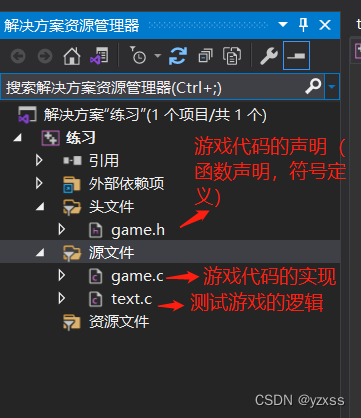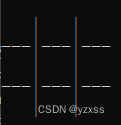# 井字棋小游戏

`最终效果如图：`# 游戏实现

## 一、创建新项目及游戏文件

1.打开VS创建新的空项目。
2.在解决方案资源管理器选中 源文件 > 右键点击添加 > 新建项 > 选中C++文件并分别创建 game.ctext.c
3.用同样的方法在解决方案资源管理器 创建头文件 game.h## 二、引头文件，定义符号

`game.h`

``````#pragma once
#define _CRT_SECURE_NO_WARNINGS
#define ROW 3 //棋盘行数
#define COL 3 //棋盘列数
#include <stdio.h>
#include<stdlib.h>
#include<time.h>
#include<windows.h>
``````

`game.c`

``````#include"game.h"
``````

`text.c`

``````#include"game.h"
``````

## 三、主函数

`text.c`

``````int main()
{

srand((unsigned int)time(NULL));//设置随机数起点
int input = 0;//接收玩家输入的数字
do
{

scanf("%d", &input);
switch (input)
{

case 0:
printf("退出成功！\n");
break;
case 1:
system("cls");//清屏
printf("开始游戏！\n\n\n");
playgame();//开始游戏
break;
default:
printf("输入错误，请重试！\n");
break;
}
} while (input);//当input为0，相当于假 退出循环
return 0;
}
``````

### 打印菜单

`game.c`

``````void printmenu()
{

printf("■■■■■■■■■■■■■■■\n");
printf("■■■    1.开始游戏    ■■■\n");
printf("■■■    0.退出游戏    ■■■\n");
printf("■■■■■■■■■■■■■■■\n");
printf("请输入数字‘0’或数字‘1’>>>\n");
}
``````

`game.h`

``````void printmenu();
``````

## 四、开始游戏

``````void playgame()
{

}
``````

### 1.创建二维数组

`text.c > playgame()函数内`

``````char table[ROW][COL]={
0};//创建一个3行3列的二维数组
``````

### 2.初始化棋盘

`game.c`

``````void inittable(char table[ROW][COL]) //初始化棋盘
{

for (int i = 0; i < ROW; i++)
{

for (int j = 0; j < COL; j++)
{

table[i][j] = ' '; //将信息置为空
}
}
}
``````

`game.h`

``````void printmenu();//打印菜单
``````

### 3.打印棋盘`game.c`

``````//打印棋盘
void printtable(char table[ROW][COL])
{

for (int i = 0; i < ROW; i++)
{

//打印空格 棋子 空格 | 空格 棋子 空格 | 空格 棋子 空格
for (int j = 0; j < COL; j++)
{

printf(" %c ", table[i][j]);
if (j < COL - 1)
{

printf("|");
}
}
printf("\n");

//打印 横杠 横杠 横杠 | 横杠 横杠 横杠 | 横杠 横杠 横杠
if (i < ROW - 1)
{

for (int j = 0; j < COL; j++)
{

printf("---");
if (j < COL - 1)
{

printf("|");
}
}
}
printf("\n");
}
}
``````

`game.h`

``````void printtable(char table[ROW][COL]);//打印棋盘
``````

### 4.玩家下棋

`game.c`

``````//玩家下棋
void player(char table[ROW][COL])
{

printf("玩家(O)走>>>> (输入: 行 空格 列 ) \n");
int row = 0;
int col = 0;
int k = 0;
while (1)
{

scanf("%d %d", &row,&col);
if (row > 0 && row <= ROW && col>0 && col<=COL && table[row - 1][col - 1] == ' ')
{

table[row-1][col-1] = 'O';
break;
}
else
{

printf("输入错误，请重试\n");
continue;
}
}
}
``````

`game.h`

``````void computer(char table[ROW][COL]);//电脑下棋
``````

### 5.电脑下棋

``````int main()
{

srand((unsigned int)time(NULL));
return 0;
}
``````

`game.c`

``````//电脑下棋
void computer(char table[ROW][COL])
{

printf("电脑(X)走>>>>\n");
while (1)
{

int col = rand() % COL;
int row = rand() % ROW;
if (table[row][col] != ' ')
{

continue;
}
else
{

table[row][col] = 'X';
break;
}
}
Sleep(500);
}
``````

`game.h`

``````void computer(char table[ROW][COL]);//电脑下棋
``````

### 6.判断游戏是否结束

check（） 函数如何判断一方的胜利呢？其实只需要分别检测井字棋的三行三列以及两个斜线八条连线 对应的三个元素是否相同，如果相同，返回对应元素的字符，如返回‘O’ 则知道是玩家赢了。如果八条连线对应的元素没有三个都相同，且已经没有空位了，返回字符‘P’表示平局。如果还有空格则返回字符‘C’表示游戏继续。

`game.c`

``````//检测是否游戏结束
char check(char table[ROW][COL])
{

int i = 0;
int j = 0;

for (j = 0; j < COL; j++)
{

if (table[j] == table[j] && table[j] == table[j] && table[j] != 0)//判断竖
{

return table[j];
}
}

for (i = 0; i < ROW; i++)
{

if (table[i] == table[i] && table[i] == table[i] && table[i] != 0)//判断横
{

return table[i];
}
}

if (table == table && table == table && table != 0)//判断斜行
{

return table;
}

if (table == table && table == table && table != 0)//判断斜行
{

return table;
}

for (i = 0; i < ROW; i++)   //判断是否继续 不是两方胜利也不是平局
{

for (j = 0; j < COL; j++)
{

if (table[i][j] == ' ')
{

return 'C';
}
}
}
return 'P'; //判断平局
}

//检测是胜利还是失败还是平局还是继续游戏。
int iswin(char table[ROW][COL])
{

char ret = check(table);
if (ret == 'O')
{

printf("恭喜你赢了！玩家胜利\n");
return 1;
}
else if (ret == 'X')
{

printf("很遗憾你输了！电脑胜利\n");
return 1;
}
else if (ret == 'P')
{

printf("平局！！！");
return 1;
}
else
{

return 0;
}
}
``````

`game.h`

``````//检测是否游戏结束
char check(char table[ROW][COL]);

//检测是胜利还是失败还是平局还是继续游戏
int iswin(char table[ROW][COL]);
``````

### 7.playgame完整函数

``````void playgame()
{

char table[ROW][COL] = {
0 };
inittable(table);//初始化棋盘
printtable(table);//打印棋盘

//进入游戏循环
while (1)
{

player(table);//玩家下棋
system("cls");//清屏
printtable(table);//打印棋盘
if (iswin(table))//检测是否游戏结束
{

system("pause");
system("cls");//清屏
break;
}

computer(table);//电脑下棋
system("cls");//清屏
printtable(table);//打印棋盘
if (iswin(table))//检测是否游戏结束
{

system("pause");
system("cls");//清屏
break;
}
}
}
``````

# 完整代码

`game.h`

``````#pragma once
#define _CRT_SECURE_NO_WARNINGS
#define ROW 3 //棋盘行数
#define COL 3 //棋盘列数
#include <stdio.h>
#include<stdlib.h>
#include<time.h>
#include<windows.h>

//打印菜单

//初始化棋盘
void inittable(char table[ROW][COL]);

//打印棋盘
void printtable(char table[ROW][COL]);

//玩家下棋
void player(char table[ROW][COL]);

//电脑下棋
void computer(char table[ROW][COL]);

//检测是否游戏结束
char check(char table[ROW][COL]);

//检测是胜利还是失败还是平局还是继续游戏
int iswin(char table[ROW][COL]);
``````

`game.c`

``````#include"game.h"

//打印菜单
{

printf("■■■■■■■■■■■■■■■\n");
printf("■■■    1.开始游戏    ■■■\n");
printf("■■■    0.退出游戏    ■■■\n");
printf("■■■■■■■■■■■■■■■\n");
printf("请输入数字‘0’或数字‘1’>>>\n");
}

//初始化棋盘
void inittable(char table[ROW][COL])
{

for (int i = 0; i < ROW; i++)
{

for (int j = 0; j < COL; j++)
{

table[i][j] = ' ';
}
}
}

//打印棋盘
void printtable(char table[ROW][COL])
{

for (int i = 0; i < ROW; i++)
{

for (int j = 0; j < COL; j++)
{

printf(" %c ", table[i][j]);
if (j < COL - 1)
{

printf("|");
}
}
printf("\n");
if (i < ROW - 1)
{

for (int j = 0; j < COL; j++)
{

printf("---");
if (j < COL - 1)
{

printf("|");
}
}
}
printf("\n");
}
}

//玩家下棋
void player(char table[ROW][COL])
{

printf("玩家(O)走>>>> (输入: 行 空格 列 ) \n");
int row = 0;
int col = 0;
int k = 0;
while (1)
{

scanf("%d %d", &row,&col);
if (row > 0 && row <= ROW && col>0 && col<=COL && table[row - 1][col - 1] == ' ')
{

table[row-1][col-1] = 'O';
break;
}
else
{

printf("输入错误，请重试\n");
continue;
}
}
}

//电脑下棋
void computer(char table[ROW][COL])
{

printf("电脑(X)走>>>>\n");
while (1)
{

int col = rand() % COL;
int row = rand() % ROW;
if (table[row][col] != ' ')
{

continue;
}
else
{

table[row][col] = 'X';
break;
}
}
Sleep(500);
}

char check(char table[ROW][COL])
{

int i = 0;
int j = 0;

for (j = 0; j < COL; j++)
{

if (table[j] == table[j] && table[j] == table[j] && table[j] != 0)//判断竖
{

return table[j];
}
}

for (i = 0; i < ROW; i++)
{

if (table[i] == table[i] && table[i] == table[i] && table[i] != 0)//判断横
{

return table[i];
}
}

if (table == table && table == table && table != 0)//判断斜行
{

return table;
}

if (table == table && table == table && table != 0)//判断斜行
{

return table;
}

for (i = 0; i < ROW; i++)   //判断是否继续 不是两方胜利也不是平局
{

for (j = 0; j < COL; j++)
{

if (table[i][j] == ' ')
{

return 'C';
}
}
}
return 'P'; //判断平局
}

int iswin(char table[ROW][COL])
{

char ret = check(table);
if (ret == 'O')
{

printf("恭喜你赢了！玩家胜利\n");
return 1;
}
else if (ret == 'X')
{

printf("很遗憾你输了！电脑胜利\n");
return 1;
}
else if (ret == 'P')
{

printf("平局！！！");
return 1;
}
else
{

return 0;
}
}
``````

`text.c`

``````#include"game.h"

void playgame()
{

char table[ROW][COL] = {
0 };
inittable(table);//初始化棋盘
printtable(table);//打印棋盘

//进入游戏循环
while (1)
{

player(table);//玩家下棋
system("cls");//清屏
printtable(table);//打印棋盘
if (iswin(table))//检测是否游戏结束
{

system("pause");
system("cls");//清屏
break;
}

computer(table);//电脑下棋
system("cls");//清屏
printtable(table);//打印棋盘
if (iswin(table))//检测是否游戏结束
{

system("pause");
system("cls");//清屏
break;
}
}
}

int main()
{

srand((unsigned int)time(NULL));
int input = 0;
do
{

scanf("%d", &input);
switch (input)
{

case 0:
printf("退出成功！\n");
break;
case 1:
system("cls");
printf("开始游戏！\n\n\n");
playgame();
break;
default:
printf("输入错误，请重试！\n");
break;
}
} while (input);
return 0;
}
``````

### SHA1算法实现及详解_Y-peak的博客-程序员秘密_sha1算法

SHA1算法实现及详解SHA1算法详解SHA1算法是Hash算法的一种。SHA1算法的最大输入长度小于2^64比特的消息，输入消息（**明文**）以512比特的分组为单位处理，输出160比特的消息摘要（**密文**）源代码#include &lt;stdio.h&gt;#include &lt;stdlib.h&gt;#include &lt;string.h&gt;#pragma warning(disable:4996)/*定义用到的常量*/unsigned int A = 0x6

### TCP三次握手的原理及攻击手段_weixin_33953384的博客-程序员秘密

TCP/IP是很多的不同的协议组成，实际上是一个协议组， TCP用户数据报表协议(也称作TCP传输控制协议，Transport Control Protocol。可靠的主机到主机层协议。这里要先强调一下，传输控制协议是OSI网络的第四层的叫法，TCP传输控制协议是TCP/IP传输的6个基本协议的一种。两个TCP意思非相同。 )。TCP是一种可靠的面向连接...